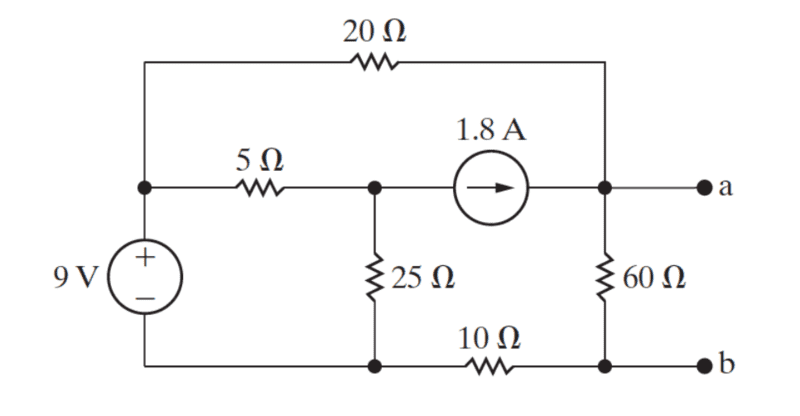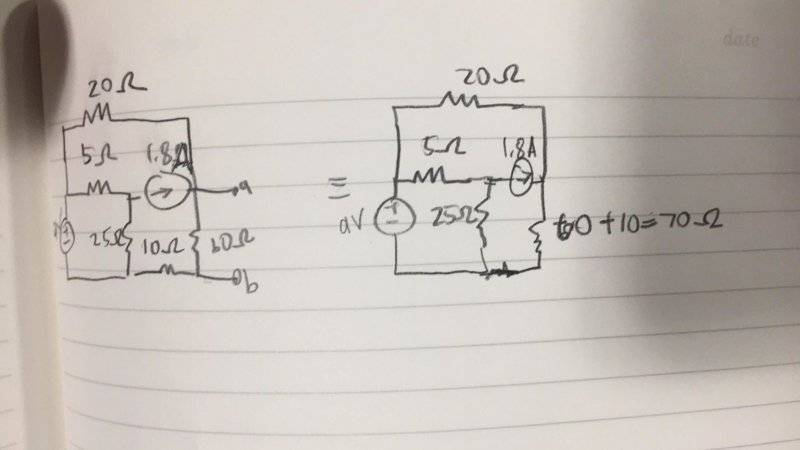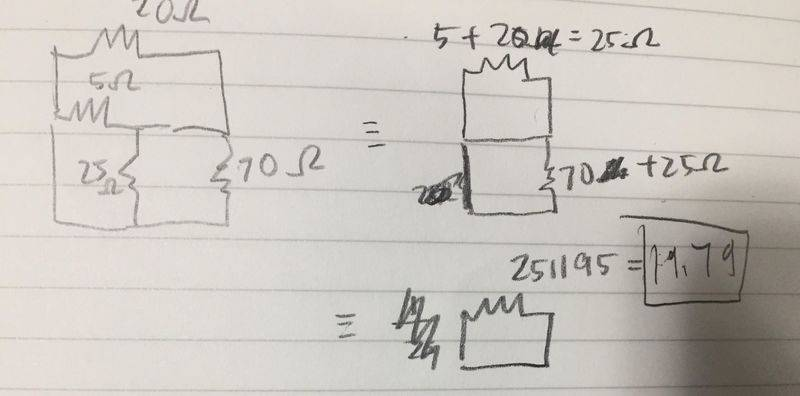# Find the Norton equivalent resistance

messier992

## Homework Statement

Find the Norton equivalent resistance## Homework Equations

Sum of resistance in a parallel circuit: 1/Rt = 1/R1 + 1/R2...
Sum of resistance a series circuit: Rt= R1+R2...

## The Attempt at a Solution

Don't know where to go from here:#### Attachments